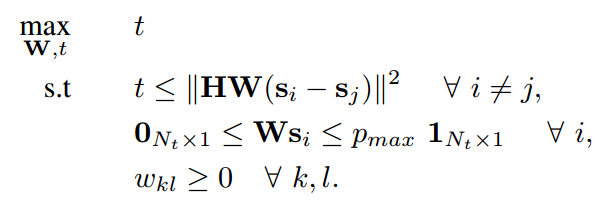# How to solve Disciplined convex programming error: Invalid constraint: {real affine} <= {convex}

Dear all,

I want to write a code to solve the following optimal problem with matlab.however, I encountered the some errors when I ran my code, Could anyone can help me figure out this problems? Please let me know if you want any further information. Thanks in advance.

clear all
close all
clc
N = 4;
Pmax = 5.ones(N,16);
O_vec=zeros(N,16);
H=10^(-5).
[0.6250 0.6101 0.5958 0.6101;0.6101 0.6250 0.6101 0.5958;0.5958 0.6101 0.6250 0.6101;0.6101 0.5958 0.6101 0.6250];
C_SMP=[0,1];
cloum=1;
for i1=1:2
for i2=1:2
for i3=1:2
for i4=1:2
ss=[C_SMP(i1);C_SMP(i2);C_SMP(i3);C_SMP(i4)];
s(:,cloum)=ss;
cloum=cloum+1;
end
end
end
end

cvx_begin quiet
variable W(N, N)
variable t
obj = t
maximize(obj)
subject to
O_vec <= W*s <= Pmax
for i=1:4
for j=1:4
if i==j
W(i,j) > 0
else
W(i,j) == 0
end
end
end

``````     for ii=1:16
s_flag=s;
s1=s_flag(:,ii);
s_flag(:,ii)=[];
for jj=1:15
s2=s_flag(:,jj);
data=norm( (H*W*s1-H*W*s2), 2 );
t <= data
end
end
``````

cvx_end
W

Disciplined convex programming error:
Invalid constraint: {real affine} <= {convex}